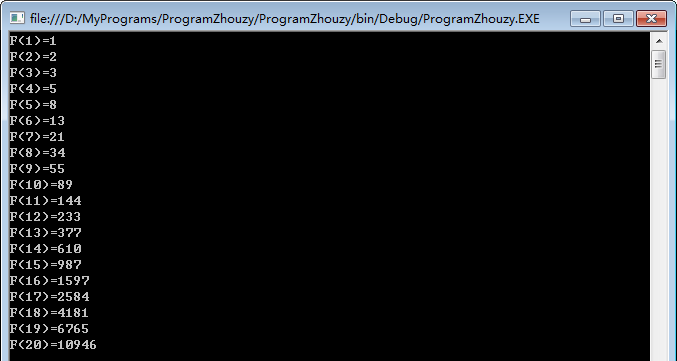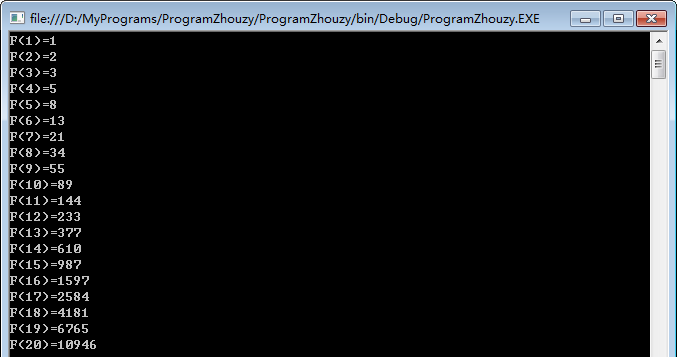# 也来说说电影《少年班》中周知庸问王大法的问题

2016/06/08 17:44（图1）（图2）

Public Function Func(x As Integer) As Integer
If x < 0 Then
Return 0
ElseIf x = 1 Then
Return 1
ElseIf x = 2 Then
Return 2
Else
Return Func(x - 1) + Func(x - 2)
End If
End FunctionF(20)=10946，电影中王大法给出的结果与之一致。

∵n>=3时，有 F(n)=F(n-1)-F(n-2)

∴n>=3时，F(n)-F(n-1)-F(n-2)=0（图3）（图4）（图5）

q1和q2都是我们所求数列的解，我们所求数列的通项公式可以写成下面的形式：（图6）（图7）（图8）（图9）（图10）

Public Function Func2(x As Integer) As Decimal
If x < 0 Then
Return 0
ElseIf x = 1 Then
Return 1
ElseIf x = 2 Then
Return 2
Else
Dim result As Decimal = 0
Dim sqrt5 As Decimal = Math.Sqrt(5)
result = ((5 + sqrt5) / 10) * ((1 + sqrt5) / 2) ^ x + _
((5 - sqrt5) / 10) * ((1 - sqrt5) / 2) ^ x
Return Math.Round(result)
End If
End FunctionEND

2
7 收藏

### 作者的其它热门文章2016/06/13 11:24

1 评论
7 收藏
2